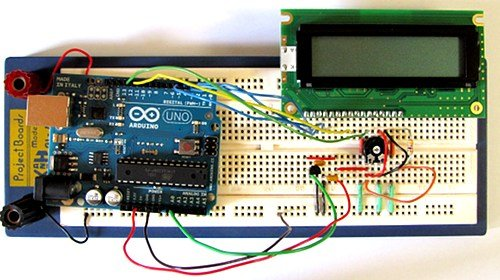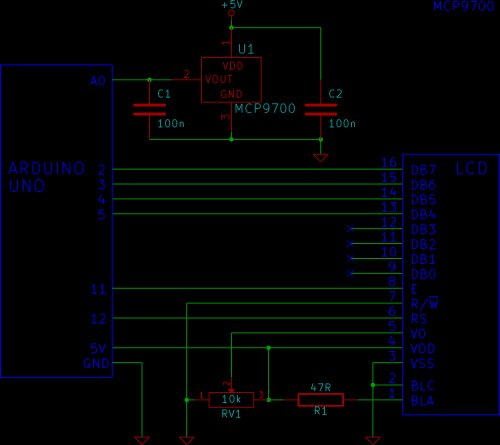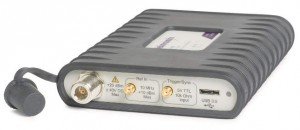# Tutorial 14: Arduino LCD Thermometer

In this tutorial, a temperature sensor (MCP9700 linear active thermistor IC) and LCD are connected to the Arduino. The Arduino reads the temperature from the MCP9700 on analog pin A0 and displays the temperature on the LCD.

This video shows the circuit operating. When touched with a finger, the temperature sensor IC will measure an increasing temperature which is displayed on the LCD.

Also see the Arduino serial thermometer tutorial (Tutorial 15).

## Prerequisites

Complete Tutorial 12: Arduino LCD before attempting this tutorial.

##(adsbygoogle = window.adsbygoogle || []).push({}); Components

Besides an Arduino Uno board, USB cable, wire links and a breadboard, you will need:

## Circuit Diagram

The MCP9700 IC is housed in a TO-92 package (it looks like a transistor). The pinout of the package is shown in the circuit diagram. Capacitors C1 and C2 are used to stabilise the output from the MCP9700 and stabilise the power to the MCP9700.

## Building the Circuit

Follow tutorial 12 to interface the LCD to the Arduino. Connect the MCP9700 to the Arduino A0 pin as shown in the circuit diagram (pin 2 of the MCP9700 connects to A0 on the Arduino). Pin 1 of the MCP9700 (marked VDD) is connected to the Arduino 5V pin via the breadboard. The GND pin of the MCP9700 is connected to one of the Arduino GND pins.

Click the picture below for a bigger image of the breadboard circuit.

## Programming the Arduino

Copy the sketch for the Arduino LCD tutorial (below) and paste it into the Arduino IDE.
/*————————————————————–
Program: LCD_temperature

Description: Reads the voltage from a MCP9700 temperature
sensor on pin A0 of the Arduino. Converts the
voltage to a temperature and displays it on an
LCD (16 character by two line).

Date: 15 April 2012

Author: W.A. Smith, http://startingelectronics.org
————————————————————–*/
#include <LiquidCrystal.h>

// Arduino pins used for LCD
LiquidCrystal lcd(12, 11, 5, 4, 3, 2);

void setup() {
// initialize the LCD display
lcd.begin(16, 2);
}

void loop() {
float temperature = 0.0; // stores the calculated temperature
int sample; // counts through ADC samples
float ten_samples = 0.0; // stores sum of 10 samples

// take 10 samples from the MCP9700
for (sample = 0; sample < 10; sample++) {
// convert A0 value to temperature
temperature = ((float)analogRead(A0) * 5.0 / 1024.0) – 0.5;
temperature = temperature / 0.01;
// sample every 0.1 seconds
delay(100);
// sum of all samples
ten_samples = ten_samples + temperature;
}// get the average value of 10 temperatures
temperature = ten_samples / 10.0;
// display the temperature on the LCD
lcd.setCursor(0, 0);
lcd.print(temperature);
lcd.print(” deg. C “);
ten_samples = 0.0;}

## Operating the Circuit

With the LCD_temperature sketch loaded into the Arduino, the temperature should be displayed on the top line of the LCD. Touching the MCP9700 temperature sensor will cause the displayed temperature to increase if the ambient temperature is below the temperature of your fingers. The temperature is displayed in degrees Celsius (°C).

For more detail: Tutorial 14: Arduino LCD Thermometer

#### This Post / Project can also be found using search terms:

• mcp9700 sensor battery life arduino
READ  DIY Android Home Automation Box